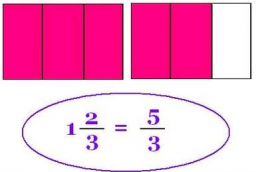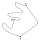# Fractions and mixed numerals

(a) Convert the following mixed numbers to improper fractions. i. 3 5/8 ii. 7 7/6 (b) Convert the following improper fraction to a mixed number. i. 13/4 ii. 78/5 (c) Simplify these fractions to their lowest terms. i. 36/42 ii. 27/45 2. evaluate the following expressions with fractions and mixed numerals: i. 1/2 - 3/5 + 4/7= ii. 1/2 ÷ 3/5 × 4/7= iii. (4/7 + 1/2) ÷ 5 3/7= iv. 2/5 × 2 1/3 + 3/2= v. 20 - (4 × 2/7 ÷ 2/21)

a1 =  3.625
a2 =  8.1667
b1 =  3.25
b2 =  15.6
c1 =  0.8571
c2 =  0.6
d1 =  0.4714
d2 =  0.4762
d3 =  0.1974
d4 =  2.4333
d5 =  8

### Step-by-step explanation:

${b}_{1}=\frac{13}{4}=\frac{13}{4}=3\frac{1}{4}=3.25$
${b}_{2}=\frac{78}{5}=\frac{78}{5}=15\frac{3}{5}=15.6$
${c}_{1}=\frac{36}{42}=\frac{6}{7}=0.8571$
${c}_{2}=\frac{27}{45}=\frac{3}{5}=0.6$
${d}_{1}=\frac{1}{2}-\frac{3}{5}+\frac{4}{7}=\frac{33}{70}=0.4714$
${d}_{3}=\left(\frac{4}{7}+\frac{1}{2}\right)\mathrm{/}5\frac{3}{7}=\frac{15}{76}=0.1974$Did you find an error or inaccuracy? Feel free to write us. Thank you!Tips to related online calculators
Need help to calculate sum, simplify or multiply fractions? Try our fraction calculator.

## Related math problems and questions:

• The rawThe raw data presented here are the scores (out of 100 marks) of a market survey regarding the acceptability of new product launched by a company for a random sample of 50 respondents: 40 45 41 45 45 30 30 8 48 25 26 9 23 24 26 29 8 40 41 42 39 35 18 25 3
• Evaluate - lowest termsEvaluate: 16/25 - 11/25 (Express answer as a fraction reduced to lowest terms. )
• Lowest termsReduce to the lowest terms: 32/124
• N percentille problemHere is a data set (n=117) that has been sorted. 10.4 12.2 14.3 15.3 17.1 17.8 18 18.6 19.1 19.9 19.9 20.3 20.6 20.7 20.7 21.2 21.3 22 22.1 22.3 22.8 23 23 23.1 23.5 24.1 24.1 24.4 24.5 24.8 24.9 25.4 25.4 25.5 25.7 25.9 26 26.1 26.2 26.7 26.8 27.5 27.6 2
• Evaluate - order of opsEvaluate the expression: 32+2[5×(24-6)]-48÷24 Pay attention to the order of operation including integers
• Evaluate mixed expressionsWhich of the following is equal to 4 and 2 over 3 divided by 3 and 1 over 2? A. 4 and 2 over 3 times 3 and 2 over 1 B. 14 over 3 times 2 over 7 C. 14 over 3 times 7 over 2 D. 42 over 3 times 2 over 31
• To improper fractionChange mixed number to improper fraction a) 1 2/15 b) -2 15/17
• Evaluate valueEvaluate value of the following expressions: 1. The product of nine and a number 12, decreased by six 2. Eight decreased by three times a number 2. 3. The quotient of twice a number 49 and seven.
• TrianglesFind out whether given sizes of the angles can be interior angles of a triangle: a) 23°10',84°30',72°20' b) 90°,41°33',48°37' c) 14°51',90°,75°49' d) 58°58',59°59',60°3'
• 6/24 change6/24 change to a fraction in lowest terms
• Speed of Slovakian trainsRudolf decided to take the train from the station 'Ostratice' to 'Horné Ozorovce'. In the train timetables found train Os 5409 : km 0 Chynorany 15:17 5 Ostratice 15:23 15:23 8 Rybany 15:27 15:27 10 Dolné Naštice 15:31 15:31 14 Bánovce nad Bebravou 15:35 1
• Equivalent fractions 2Write the equivalent multiplication expression. 2 1/6÷3/4
• Evaluate 17Evaluate 2x+6y when x=- 4/5 and y=1/3. Write your answer as a fraction or mixed number in simplest form.
• The machineThe machine was in operation for 9 tenths (in a fraction) of eight hours of working time. How long has he been running?
• Reducing scaleI am sizing some landscaping and I measured everything for a 20 to 1 scale. I realized when I was done that it was at 30 to 1. How can I convert what I already have to 30 to 1. (Example, grass area is 22,871.6' at 20 to 1, how do you figure it out to be 3
• Simplify 3Simplify mixed numerals expression: 8 1/4- 3 2/5 - (2 1/3 - 1/4) Show your solution.
• RemaindersIt is given a set of numbers { 170; 244; 299; 333; 351; 391; 423; 644 }. Divide this numbers by number 66 and determine set of remainders. As result write sum of this remainders.